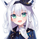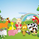2793 lượt xem
Return a linear regression channel with a window size within the range (min, max) such that the R-squared is maximized, this allows a better estimate of an underlying linear trend, a better detection of significant historical supports and resistance points, and avoid finding a good window size manually.

Settings

• Min : Minimum window size value
• Max : Maximum window size value
• Mult : Multiplicative factor for the rmse, control the channel width.
• Src : Source input of the indicator

Details

The indicator displays the specific window size that maximizes the R-squared at the bottom of the lower channel.

When optimizing we want to find parameters such that they maximize or minimize a certain function, here the r-squared . The R-squared is given by 1 minus the ratio between the sum of squares ( SSE ) of the linear regression and the sum of squares of the mean. We know that the mean will always produce an SSE greater or equal to the one of the linear regression , so the R-squared will always be in a (0,1) range. In the case our data has a linear trend, the linear regression will have a better fit, thus having a lower SSE than the SSE of the mean, has such the ratio between the linear regression SSE and the mean SSE will be low, 1 minus this ratio will return a greater result. A lower R-squared will tell you that your linear regression produces a fit similar to the one produced by the mean. The R-squared is also given by the square of the correlation coefficient between the dependent and independent variables.

In pinescript optimization can be done by running a function inside a loop, we run the function for each setting and keep the one that produces the maximum or minimum result, however, it is not possible to do that with most built-in functions, including the function of interest, correlation, as such we must recreate a rolling correlation function that can be used inside loops, such functions are generally loops-free, this means that they are not computed using a loop in the first place, fortunately, the rolling correlation function is simply based on moving averages and standard deviations, both can be computed without using a loop by using cumulative sums, this is what is done in the code.

Note that because the R-squared is based on the SSE of the linear regression , maximizing the R-squared also minimizes the linear regression SSE , another thing that is minimized is the horizontality of the fit.

In the example above we have a total window size of 27, the script will try to find the setting that maximizes the R-squared , we must avoid every data points before the volatile bearish candle, using any of these data points will produce a poor fit, we see that the script avoid it, thus running as expected. Another interesting thing is that the best R-squared is not always associated to the lowest window size.

Note that optimization does not fix core problems in a model, with the linear regression we assume that our data set posses a linear trend, if it's not the case, then no matter how many settings you use you will still have a model that is not adapted to your data.
Patreon: https://www.patreon.com/alexgrover